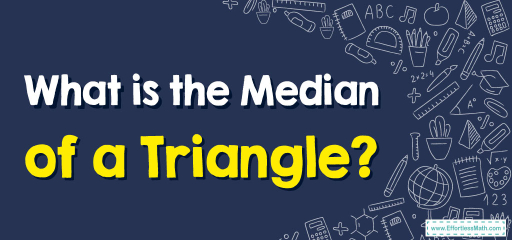# What is the Median of a Triangle?

The median of a triangle, often introduced to students in early geometry, is more than just a line segment. It connects a vertex of the triangle to the midpoint of the opposite side, providing insight into the triangle's internal structure and balance. As one of the triangle's notable centers, understanding the median is essential for deeper explorations into triangle properties and centroid-related concepts. This guide aims to shed light on the intricacies of the median, its significance, and its role in various geometric applications.## Step-by-step Guide: A Median of a Triangle

1. Definition of a Median:
A median of a triangle is a line segment that connects a vertex of the triangle to the midpoint of the opposite side. Each triangle has three medians, one from each vertex, and they all intersect at a single point called the centroid.

2. Properties of a Median:

• Centroid Division: The centroid divides each median into two segments, with the segment connecting the centroid to the vertex being twice as long as the segment connecting the centroid to the midpoint of the side.
• Concurrent Medians: All three medians of a triangle are concurrent, meaning they meet at one point – the centroid. This point also serves as the triangle’s center of gravity.
• Area Relation: The medians of a triangle divide it into six smaller triangles of equal area.

### Examples

Example 1:
Given a triangle with vertices $$A$$, $$B$$, and $$C$$. If the median from vertex $$A$$ to side $$BC$$ measures $$12 \text{ cm}$$ and the centroid is located $$8 \text{ cm}$$ away from $$A$$, determine the length from the centroid to side $$BC$$.

Solution:
Using the property of median division by the centroid:
The segment from $$A$$ to the centroid is twice as long as the segment from the centroid to the midpoint of $$BC$$.
Given that the distance from $$A$$ to the centroid is $$8 \text{ cm}$$, the distance from the centroid to the midpoint of $$BC$$ is half of that, which is $$4 \text{ cm}$$.

Example 2:
In a triangle with vertices $$P$$, $$Q$$, and $$R$$, if the median from vertex $$P$$ to side $$QR$$ has a length of $$15 \text{ cm}$$, and the centroid divides this median in the ratio $$2:1$$, find the lengths of the segments into which the median is divided.

Solution:
Using the $$2:1$$ division ratio of the median by the centroid:
Length from P to centroid $$= \frac{2}{3} \times 15 \text{ cm} = 10 \text{ cm}$$
Length from centroid to midpoint of QR $$= \frac{1}{3} \times 15 \text{ cm} = 5 \text{ cm}$$

### Practice Questions:

1. If a median of a triangle is $$18 \text{ cm}$$ long, how far is the centroid from the triangle’s vertex?
2. In a triangle, the distance from a vertex to the centroid along a median is $$9 \text{ cm}$$. What is the total length of this median?

1. $$\frac{2}{3} \times 18 \text{ cm} = 12 \text{ cm}$$
2. Given the $$2:1$$ ratio, the total median length $$= 9 \text{ cm} \times 3 = 27 \text{ cm}$$

### What people say about "What is the Median of a Triangle? - Effortless Math: We Help Students Learn to LOVE Mathematics"?

No one replied yet.

X
30% OFF

Limited time only!

Save Over 30%

SAVE $5 It was$16.99 now it is \$11.99